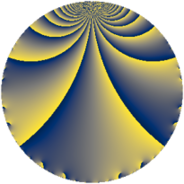Properties

 Label 6720.2.a.cwLevel $6720$ Weight $2$ Character orbit 6720.a Self dual yes Analytic conductor $53.659$ Analytic rank $0$ Dimension $2$ CM no Inner twists $1$

Related objects

Newspace parameters

 Level: $$N$$ $$=$$ $$6720 = 2^{6} \cdot 3 \cdot 5 \cdot 7$$ Weight: $$k$$ $$=$$ $$2$$ Character orbit: $$[\chi]$$ $$=$$ 6720.a (trivial)

Newform invariants

 Self dual: yes Analytic conductor: $$53.6594701583$$ Analytic rank: $$0$$ Dimension: $$2$$ Coefficient field: $$\Q(\sqrt{17})$$ Defining polynomial: $$x^{2} - x - 4$$ Coefficient ring: $$\Z[a_1, \ldots, a_{11}]$$ Coefficient ring index: $$2$$ Twist minimal: no (minimal twist has level 3360) Fricke sign: $$-1$$ Sato-Tate group: $\mathrm{SU}(2)$

$q$-expansion

Coefficients of the $$q$$-expansion are expressed in terms of $$\beta = \sqrt{17}$$. We also show the integral $$q$$-expansion of the trace form.

 $$f(q)$$ $$=$$ $$q + q^{3} + q^{5} - q^{7} + q^{9} +O(q^{10})$$ $$q + q^{3} + q^{5} - q^{7} + q^{9} + ( 1 + \beta ) q^{11} + ( -1 + \beta ) q^{13} + q^{15} + 2 q^{17} + ( 3 - \beta ) q^{19} - q^{21} + q^{25} + q^{27} + 2 q^{29} + ( -1 - \beta ) q^{31} + ( 1 + \beta ) q^{33} - q^{35} + 2 q^{37} + ( -1 + \beta ) q^{39} + 2 q^{41} + ( 2 + 2 \beta ) q^{43} + q^{45} + q^{49} + 2 q^{51} + ( -1 - 3 \beta ) q^{53} + ( 1 + \beta ) q^{55} + ( 3 - \beta ) q^{57} -4 q^{59} + 2 \beta q^{61} - q^{63} + ( -1 + \beta ) q^{65} + ( 6 - 2 \beta ) q^{67} + ( 1 + \beta ) q^{71} + ( 11 + \beta ) q^{73} + q^{75} + ( -1 - \beta ) q^{77} + ( -6 + 2 \beta ) q^{79} + q^{81} -4 q^{83} + 2 q^{85} + 2 q^{87} + ( 8 - 2 \beta ) q^{89} + ( 1 - \beta ) q^{91} + ( -1 - \beta ) q^{93} + ( 3 - \beta ) q^{95} + ( 9 - \beta ) q^{97} + ( 1 + \beta ) q^{99} +O(q^{100})$$ $$\operatorname{Tr}(f)(q)$$ $$=$$ $$2 q + 2 q^{3} + 2 q^{5} - 2 q^{7} + 2 q^{9} + O(q^{10})$$ $$2 q + 2 q^{3} + 2 q^{5} - 2 q^{7} + 2 q^{9} + 2 q^{11} - 2 q^{13} + 2 q^{15} + 4 q^{17} + 6 q^{19} - 2 q^{21} + 2 q^{25} + 2 q^{27} + 4 q^{29} - 2 q^{31} + 2 q^{33} - 2 q^{35} + 4 q^{37} - 2 q^{39} + 4 q^{41} + 4 q^{43} + 2 q^{45} + 2 q^{49} + 4 q^{51} - 2 q^{53} + 2 q^{55} + 6 q^{57} - 8 q^{59} - 2 q^{63} - 2 q^{65} + 12 q^{67} + 2 q^{71} + 22 q^{73} + 2 q^{75} - 2 q^{77} - 12 q^{79} + 2 q^{81} - 8 q^{83} + 4 q^{85} + 4 q^{87} + 16 q^{89} + 2 q^{91} - 2 q^{93} + 6 q^{95} + 18 q^{97} + 2 q^{99} + O(q^{100})$$

Embeddings

For each embedding $$\iota_m$$ of the coefficient field, the values $$\iota_m(a_n)$$ are shown below.

For more information on an embedded modular form you can click on its label.

Label $$\iota_m(\nu)$$ $$a_{2}$$ $$a_{3}$$ $$a_{4}$$ $$a_{5}$$ $$a_{6}$$ $$a_{7}$$ $$a_{8}$$ $$a_{9}$$ $$a_{10}$$
1.1
 −1.56155 2.56155
0 1.00000 0 1.00000 0 −1.00000 0 1.00000 0
1.2 0 1.00000 0 1.00000 0 −1.00000 0 1.00000 0
 $$n$$: e.g. 2-40 or 990-1000 Significant digits: Format: Complex embeddings Normalized embeddings Satake parameters Satake angles

Atkin-Lehner signs

$$p$$ Sign
$$2$$ $$-1$$
$$3$$ $$-1$$
$$5$$ $$-1$$
$$7$$ $$1$$

Inner twists

This newform does not admit any (nontrivial) inner twists.

Twists

By twisting character orbit
Char Parity Ord Mult Type Twist Min Dim
1.a even 1 1 trivial 6720.2.a.cw 2
4.b odd 2 1 6720.2.a.ct 2
8.b even 2 1 3360.2.a.ba 2
8.d odd 2 1 3360.2.a.bg yes 2

By twisted newform orbit
Twist Min Dim Char Parity Ord Mult Type
3360.2.a.ba 2 8.b even 2 1
3360.2.a.bg yes 2 8.d odd 2 1
6720.2.a.ct 2 4.b odd 2 1
6720.2.a.cw 2 1.a even 1 1 trivial

Hecke kernels

This newform subspace can be constructed as the intersection of the kernels of the following linear operators acting on $$S_{2}^{\mathrm{new}}(\Gamma_0(6720))$$:

 $$T_{11}^{2} - 2 T_{11} - 16$$ $$T_{13}^{2} + 2 T_{13} - 16$$ $$T_{17} - 2$$ $$T_{19}^{2} - 6 T_{19} - 8$$ $$T_{23}$$ $$T_{29} - 2$$ $$T_{31}^{2} + 2 T_{31} - 16$$

Hecke characteristic polynomials

$p$ $F_p(T)$
$2$ $$T^{2}$$
$3$ $$( -1 + T )^{2}$$
$5$ $$( -1 + T )^{2}$$
$7$ $$( 1 + T )^{2}$$
$11$ $$-16 - 2 T + T^{2}$$
$13$ $$-16 + 2 T + T^{2}$$
$17$ $$( -2 + T )^{2}$$
$19$ $$-8 - 6 T + T^{2}$$
$23$ $$T^{2}$$
$29$ $$( -2 + T )^{2}$$
$31$ $$-16 + 2 T + T^{2}$$
$37$ $$( -2 + T )^{2}$$
$41$ $$( -2 + T )^{2}$$
$43$ $$-64 - 4 T + T^{2}$$
$47$ $$T^{2}$$
$53$ $$-152 + 2 T + T^{2}$$
$59$ $$( 4 + T )^{2}$$
$61$ $$-68 + T^{2}$$
$67$ $$-32 - 12 T + T^{2}$$
$71$ $$-16 - 2 T + T^{2}$$
$73$ $$104 - 22 T + T^{2}$$
$79$ $$-32 + 12 T + T^{2}$$
$83$ $$( 4 + T )^{2}$$
$89$ $$-4 - 16 T + T^{2}$$
$97$ $$64 - 18 T + T^{2}$$